# Amplitude balancing for AVO analysis

Arnaud Berlioux and David Lumley

Author has no known email address

# ABSTRACT

Source and receiver amplitude variations can distort AVO analysis of prestack seismic reflection data. We therefore perform an amplitude balancing of seismic traces from a marine data set. We address this problem by computing the total energy of each trace in the seismic survey, and remove the global low-wavenumber amplitude trend from the resulting 2-D trace energy map. We then estimate an amplitude coefficient for each physical hydrophone position in the recording cable, and each shot position along the survey line. We apply these coefficients to the original seismic traces and successfully remove most of the source and receiver amplitude variation.

INTRODUCTION

Ultimately, we plan to perform an AVO analysis on the data set provided by Mobil. However, some care needs to be taken in the preprocessing stages to ensure that amplitude information is correct. In particular, we address the issue of source and receiver amplitude balancing.

The marine cable used to record the Mobil data consisted of hydrophones of varying amplitude response. Some receivers are relatively weaker or stronger than others. Additionally, some of the source strengths vary along the survey line, due to varying air gun pressure and misfires. Therefore, amplitude balancing of the traces is necessary. Yu has discussed an amplitude balancing method. We assume a simple amplitude model where receiver amplitudes are constant for all shot locations at a given hydrophone position in the cable. Similarly, we assume that a source amplitude is constant for all receivers at a particular shot location.

To perform a non-biased AVO analysis, we need to estimate these source and receiver amplitude responses, and compensate the prestack traces accordingly. We first calculate the total integrated energy for each trace in the survey. Then we remove the global low-wavenumber trend of this 2-D trace energy map. Finally, we determine the source and receiver amplitude balancing coefficients for each shot and receiver, and apply them to balance the original prestack data.

AN AMPLITUDE BALANCING MODEL

In the field, acquisition systems used in seismic exploration surveys are composed essentially of a source S that generates waves and receivers Ri which are geophones on land or hydrophones in the sea.

In a marine survey, the receivers are lined up in a streamer pulled by the ship. Figure survey shows a ship performing a marine seismic survey.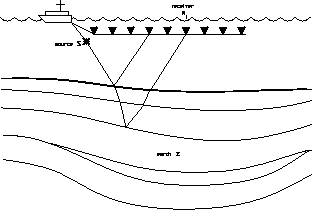survey
Figure 1
Ship pulling a streamer during a marine survey.

For a shot gather, each trace recorded at the receiver Ri can be interpreted as the result of the convolution of the source impulse wavelet S with the earth model E and with the receiver impulse response Ri: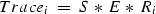(1)

Receiver impulse responses vary along the cable, some of them are stronger, others are weaker.

We want to test different AVO schemes on the Mobil data set. Because the traces recorded by some receivers have an amplitude which seems to be too different from the average response of the other receivers, it is necessary to correct for these anomalies before doing the AVO analysis, and increase or decrease the amplitude of the corresponding receivers in each shot gather. This operation is called balancing the cable'' since we want to obtain amplitudes that would have been recorded by a cable made of ideal copies of a unique receiver.

In the following two sections, we consider that the total trace energy obtained by summing the squared amplitudes along the time axis satisfy the following model: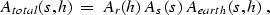(2)
where Ar is the component of the amplitude due to the receivers and depends on the offset h, As is the component of the amplitude due to the source and depends on the shot coordinate, and Aearth is the component of the amplitude due to the earth and depends on the offset and the shot coordinate. Aearth is actually the product of the structural geology and AVO components.

Taking the logarithm of expression (2), we have: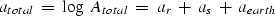(3)
where each ai is the logarithm of the corresponding Ai.

After correcting atotal for the low-wavenumber trend of Aearth, we should obtain:(4)
whereis the logarithm of the corrected amplitude corresponding to Ai, and noise represents some background noise due mostly to the high-wavenumber earth component.

REMOVING THE GLOBAL AMPLITUDE TREND

We first calculate the total integrated energy for each trace of the survey by summing the amplitudes of the traces over the time axis. Figure amplitude shows an amplitude plot as a function of offset and shot number. This amplitude plot has been obtained by taking the square root of the 2-D energy map. In Figure amp3d the same amplitude function is represented as a 2-D surface. Its shape has a global exponential decay in the offset direction and a linear trend in the shot direction.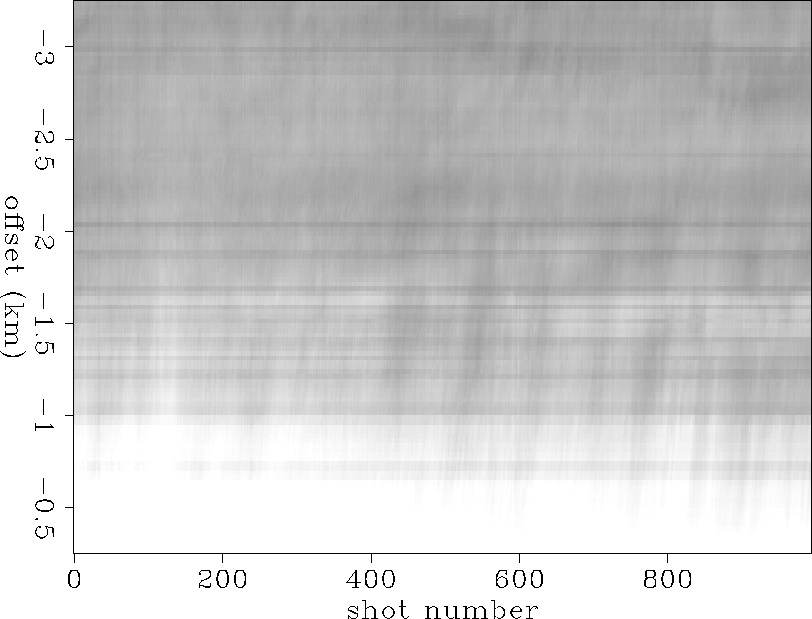amplitude
Figure 2
Amplitude plot of the Mobil data set. The stripes in the horizontal direction correspond to variations of the impulse response of some receivers, while stripes in the vertical direction can be interpreted as variations of the energy of the source.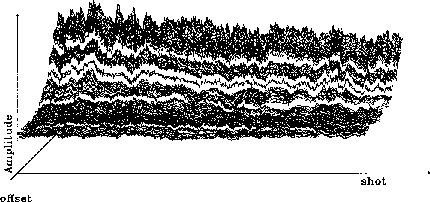amp3d
Figure 3
3-D amplitude surface showing the global trend in both directions.

The plot of the 2-D amplitude function (Figure amplitude) shows horizontal stripes parallel to the shot direction, visible especially around an offset of -1.5 km, and some less visible vertical stripes parallel to the offset direction. These horizontal and vertical lines correspond respectively to variations of the impulse response of some receivers and variations of source strength. In order to estimate the receiver and shot response, we first remove the global trend in the 2-D amplitude function.

Removing the trend in the offset direction

In the offset direction, the amplitude curve for a representative shot is plotted as the thick solid line in Figure shot120. We want to remove the global trend of this curve to reveal the residuals.

As a first approach we tried to obtain a smooth version of the 1-D amplitude curve by applying the SEP Smooth program with a four-point triangle filter, and low-pass filtering in the wavelet domain. These methods did not produce results accurate enough so far, but may be the subject of further investigation.

The general shape of these curves leads us to fit an exponential function of offset: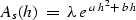(5)
where, a, and b are constants and h is the offset variable. We use a least-squares approximation to estimate the coefficients, a, and b.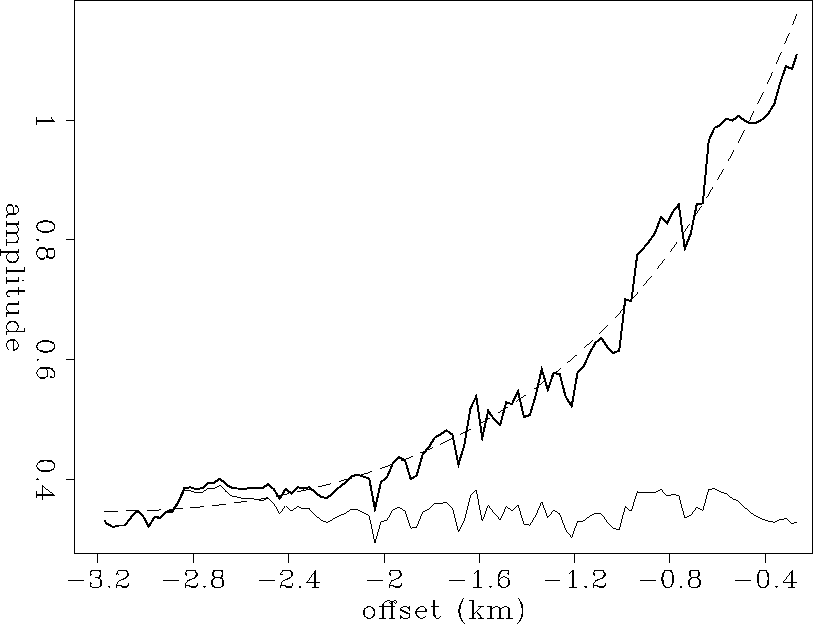shot120
Figure 4
Removal of the general trend for the amplitude curve in the offset direction. The thick solid curve represents the amplitude for shot point number 120. The dashed line is the function obtained by least-squares fitting to the data and the solid curve at the bottom of the plot is the residual after removing the exponential trend.

In order to flatten out the original amplitude curve, we multiply it by a correction coefficient: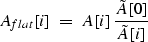(6)
where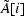is the 1-D amplitude function estimated by least-squares fitting. The scaling factor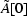has been chosen to be the far offset value of the amplitude curve because the 2-D amplitude surface is smoother on this portion of the plane, as can be seen in Figure amp3d.

Figure shot120 shows the result we obtain for the amplitude curve corresponding to shot number 120. The values of the estimated coefficients for the least-squares fitting curve are:(7)
The solid line at the bottom of the plot represents the amplitude curve after applying the correction coefficients to the original amplitude (thick solid line), as described in formula (6).

The same method has been applied to the entire 2-D amplitude plane. A best-fitting curve has been estimated in the offset direction for each shot point, and removed from the original amplitude in order to flatten out the amplitude surface in the offset direction.

Removing the trend in the shot direction

In Figure offset120, the thick curve is the amplitude for the nearest offset (offset -0.2375 km) as a function of shot coordinate. The global shape of the amplitude curves in the shot direction is less complicated than in the offset direction (see Figure amp3d). We approximated it by the linear function:(8)
whereand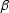are determined by a least-squares estimation. Then, the shot amplitude trend is corrected in a similar manner as the receiver trend.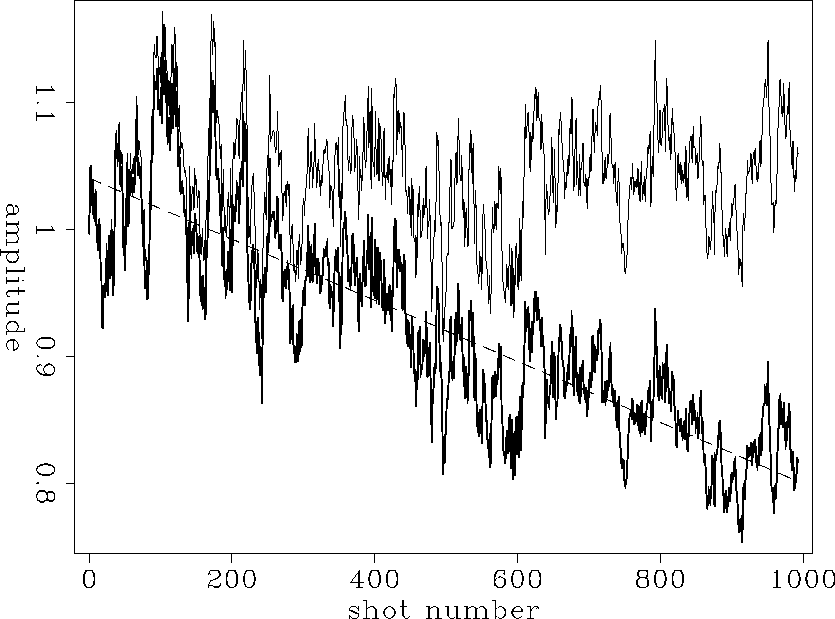offset120
Figure 5
Removal of the general trend for the amplitude curve in the shot direction. The thick continuous curve represents the amplitude for the nearest offset. The dashed dipping line has been obtained by least-squares fitting to the data and the continuous curve at the top of the plot is the amplitude flattened by removal of the dipping linear trend.

The estimated value of the coefficients are for the amplitude curve corresponding to the nearest offset the following: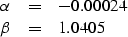(9)

This technique is then applied to the 2-D amplitude plane. A best-fitting curve has been estimated in the shot direction for each offset, and removed from the original amplitude in order to flatten out the amplitude surface in the shot direction.trend
Figure 6
2-D amplitude plot after removal of the global trend in both directions.

Figure trend shows the amplitude plane after the removal of its global trend. Removing the exponential trend in the offset direction and the linear trend in the shot direction leaves a globally flat 2-D amplitude surface with some high-frequency amplitude variation. The flattened amplitude surface presents a uniform grey background, and stripes in the receiver and shot directions have become more apparent. The grey background can be interpreted as an average response of the shots and receivers, and the component of the amplitude due to the geology as described in equation (2). The horizontal stripes correspond to receivers with an amplitude response that deviates from the global average. The vertical stripes correspond to variations of the source energy. Both of these variations have to be corrected for a subsequent AVO analysis.

AMPLITUDE BALANCING

Starting with the amplitude plane shown in Figure trend, we estimate the correction coefficients in both directions that have to be applied to the data in order to remove the variations. We stack the corrected amplitude surface in the shot direction to obtain the receiver coefficients (Figure offset-coef) and in the offset direction to obtain the shot coefficients (Figure shot-coef).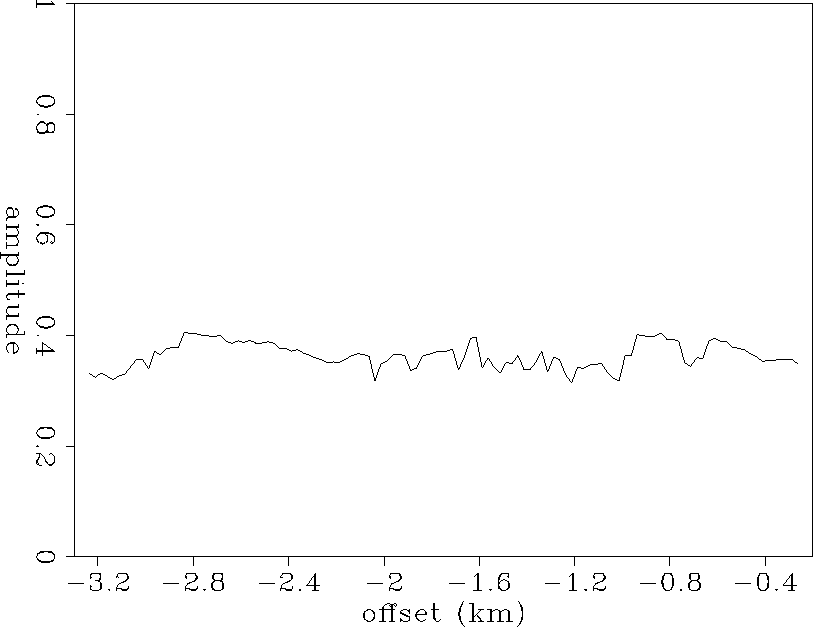offset-coef
Figure 7
Correction coefficients calculated in the offset direction.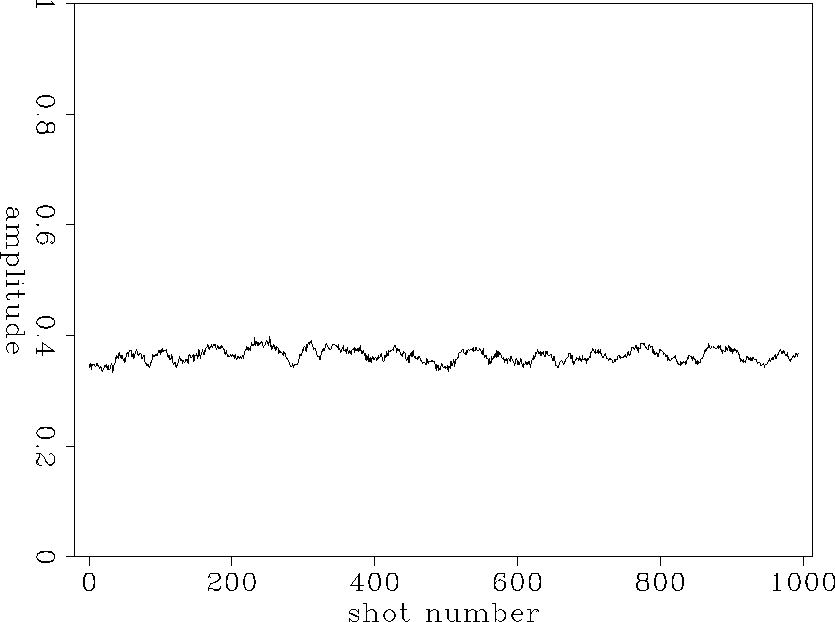shot-coef
Figure 8
Correction coefficients calculated in the shot direction.

We then use these coefficients to remove the stripes from the plot in Figure trend. Figure rem shows the amplitude plot obtained after dividing the 2-D flattened amplitude of Figure trend by the coefficients in the offset and shot directions. This shows the contribution of the earth components to the amplitude of the traces recorded during the survey. Aside from this earth contribution, some residual noise, and receiver and source variations might still be present but not dominant.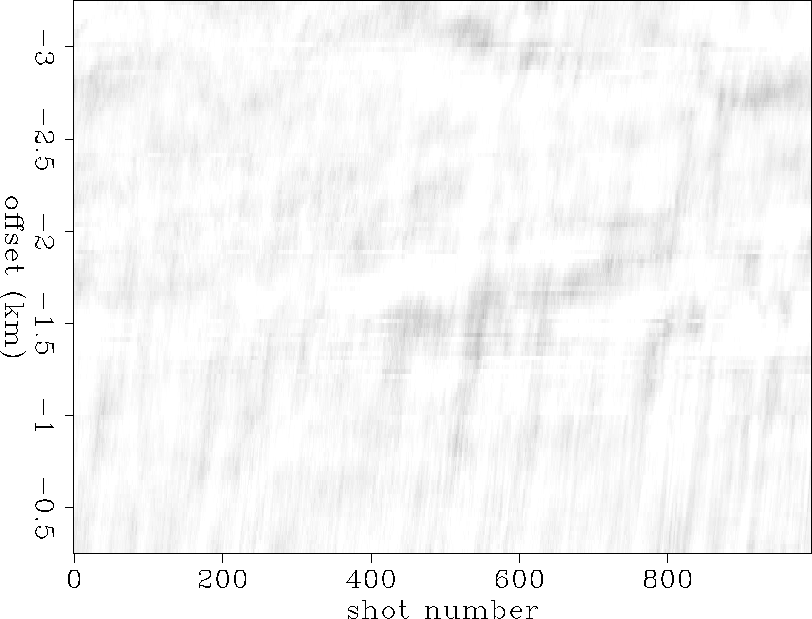rem
Figure 9
Amplitude obtained after removal of the shot and receiver stripes in the two directions.stripes
Figure 10
Plot of the shot and receiver stripes only.

Figure stripes represents only the stripes, corresponding to the plane of the combined shot and receiver amplitude coefficients, Ar(h) As(s).

CONCLUSIONS

We have assumed a simple amplitude model in which receiver amplitudes are constant at a given hydrophone position in the cable for all shot locations, and source amplitudes are constant for all receivers at a particular shot location. The method of amplitude balancing resulting from this assumption produces good results. We removed the strong variations of the receiver and source components as shown on the amplitude plots (Figure rem). Our amplitude balancing can be useful in order to perform a good AVO analysis. We plan to investigate other methods of amplitude balancing, including wavelet transforms.

[GEOTLE]